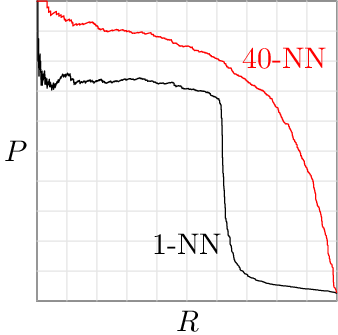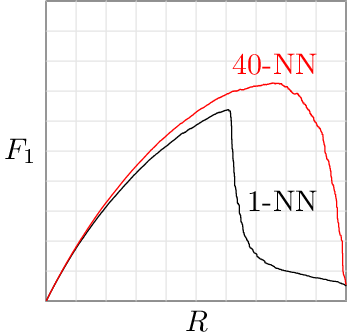# Misleading Metrics and Irrelevant Research (Accuracy and F1)

If one algorithm achieved 98.2% accuracy while another had 98.6% for the same task, would you be surprised to find that the first algorithm required ten times as much document review to reach 75% recall compared to the second algorithm?  This article explains why some performance metrics don’t give an accurate view of performance for ediscovery purposes, and why that makes a lot of research utilizing such metrics irrelevant for ediscovery.

The key performance metrics for ediscovery are precision and recall.  Recall, R, is the percentage of all relevant documents that have been found.  High recall is critical to defensibility.  Precision, P, is the percentage of documents predicted to be relevant that actually are relevant.  High precision is desirable to avoid wasting time reviewing non-relevant documents (if documents will be reviewed to confirm relevance and check for privilege before production).  In other words, precision is related to cost.  Specifically, 1/P is the average number of documents you’ll have to review per relevant document found.  When using technology-assisted review (predictive coding), documents can be sorted by relevance score and you can choose any point in the sorted list and compute the recall and precision that would be achieved by treating documents above that point as being predicted to be relevant.  One can plot a precision-recall curve by doing precision and recall calculations at various points in the sorted document list.

The precision-recall curve to the rightcompares two different classification algorithms applied to the same task.  To do a sensible comparison, we should compare precision values at the same level of recall.  In other words, we should compare the cost of reaching equally good (same recall) productions.  Furthermore, the recall level where the algorithms are compared should be one that is sensible for for ediscovery — achieving high precision at a recall level a court wouldn’t accept isn’t very useful.  If we compare the two algorithms at R=75%, 1-NN has P=6.6% and 40-NN has P=70.4%.  In other words, if you sort by relevance score with the two algorithms and review documents from top down until 75% of the relevant documents are found, you would review 15.2 documents per relevant document found with 1-NN and 1.4 documents per relevant document found with 40-NN.  The 1-NN algorithm would require over ten times as much document review as 40-NN.  1-NN has been used in some popular TAR systems.  I explained why it performs so badly in a previous article.

There are many other performance metrics, but they can be written as a mixture of precision and recall (see Chapter 7 of the current draft of my book).  Anything that is a mixture of precision and recall should raise an eyebrow — how can you mix together two fundamentally different things (defensibility and cost) into a single number and get a useful result?  Such metrics imply a trade-off between defensibility and cost that is not based on reality.  Research papers that aren’t focused on ediscovery often use such performance measures and compare algorithms without worrying about whether they are achieving the same recall, or whether the recall is high enough to be considered sufficient for ediscovery.  Thus, many conclusions about algorithm effectiveness simply aren’t applicable for ediscovery because they aren’t based on relevant metrics.

One popular metric is accuracy,which is the percentage of predictions that are correct.  If a system predicts that none of the documents are relevant and prevalence is 10% (meaning 10% of the documents are relevant), it will have 90% accuracy because its predictions were correct for all of the non-relevant documents.  If prevalence is 1%, a system that predicts none of the documents are relevant achieves 99% accuracy.  Such incredibly high numbers for algorithms that fail to find anything!  When prevalence is low, as it often is in ediscovery, accuracy makes everything look like it performs well, including algorithms like 1-NN that can be a disaster at high recall.  The graph to the right shows the accuracy-recall curve that corresponds to the earlier precision-recall curve (prevalence is 2.633% in this case), showing that it is easy to achieve high accuracy with a poor algorithm by evaluating it at a low recall level that would not be acceptable for ediscovery.  The maximum accuracy achieved by 1-NN in this case was 98.2% and the max for 40-NN was 98.6%.  In case you are curious, the relationship between accuracy, precision, and recall is:$ACC = 1 - \rho (1 - R) - \rho R (1 - P) / P$
where$\rho$ is the prevalence.

Another popular metric is the F1 score.I’ve criticized its use in ediscovery before.  The relationship to precision and recall is:$F_1 = 2 P R / (P + R)$
The F1 score lies between the precision and the recall, and is closer to the smaller of the two.  As far as F1 is concerned, 30% recall with 90% precision is just as good as 90% recall with 30% precision (both give F1 = 0.45) even though the former probably wouldn’t be accepted by a court and the latter would.   F1 cannot be large at small recall, unlike accuracy, but it can be moderately high at modest recall, making it possible to achieve a decent F1 score even if performance is disastrously bad at the high recall levels demanded by ediscovery.  The graph to the right shows that 1-NN manages to achieve a maximum F1 of 0.64, which seems pretty good compared to the 0.73 achieved by 40-NN, giving no hint that 1-NN requires ten times as much review to achieve 75% recall in this example.

Hopefully this article has convinced you that it is important for research papers to use the right metric, specifically precision (or review effort) at high recall, when making algorithm comparisons that are useful for ediscovery.